# The universe is expanding faster than the speed of light, how is it calculated?

Einstein's special theory of relativity holds that the speed of light in the universe is the fastest, and that all motion of matter cannot surpass the speed of light. However, in the standard model of the universe, the expansion of the universe exceeds the speed of light, in a way that has proved very difficult to understand, since the motion of matter cannot exceed the speed of light. the speed of light, so why is the expansion of the universe faster than the speed of light?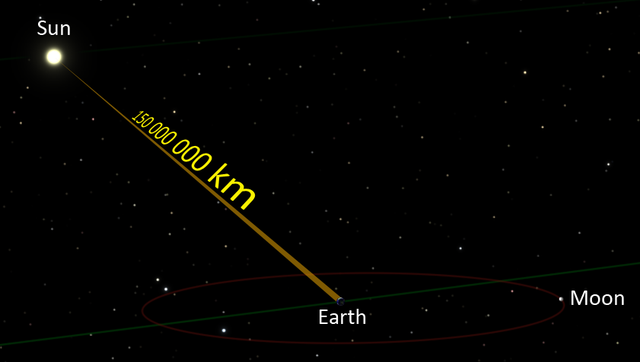In fact, the essence of the answers to these questions is the difference between the expansion of the universe faster than the speed of light and the speed of light in the motion of objects. Simply put, the speed of light barrier can only be applied to objects with static mass, which cannot exceed the speed of light, whereas the expansion of the universe is the expansion of space-time. Space-time itself has no mass, and of course it is not limited by the speed of light.

What is the expansion of the universe?

Cosmic expansion refers to the continuous expansion of the entire universe on a large scale, this was first discovered and provided by the famous American astronomer Edwin Hubble. In his memory, mankind's first modern space telescope was named after him. After the Hubble Space Telescope was put into the sky, it greatly expanded humanity's vision and deepened understanding of the universe.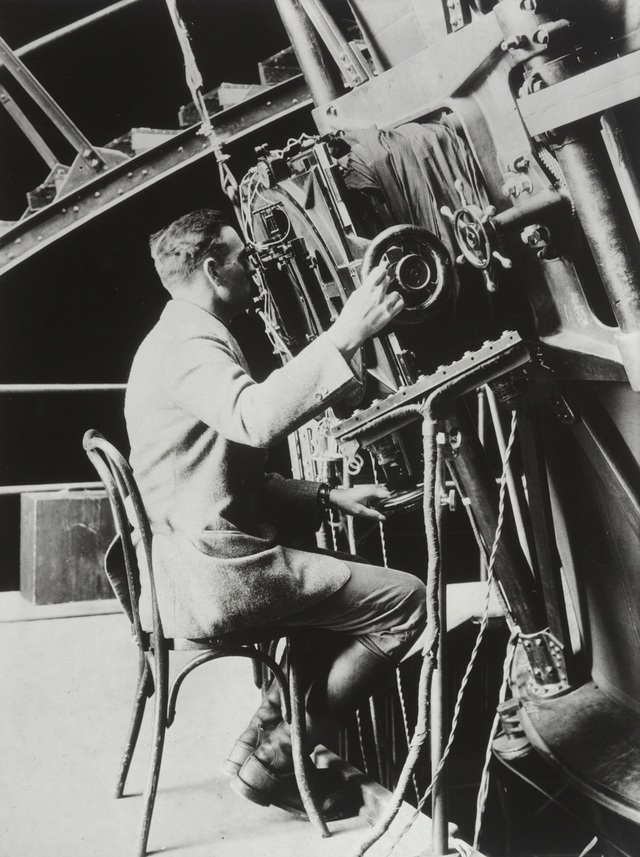Hubble came to a conclusion about the expansion of the universe in 1929 based on long-term observations. The conclusion is that the entire universe is expanding and homogenizing, all the galaxies are separate, getting further and further apart.

From this he deduced Hubble's law, which is simply expressed as: V = HD. Here V represents the rate of regress of the galaxy; H represents the Hubble constant, which is defined as the rate of regression of the galaxy at a distance of 10Mpc from us, in s/km; D represents the actual distance between the galaxy and us. Mpc is a unit of megaparsec, and 1pc (parsec) is about 3.26 light years.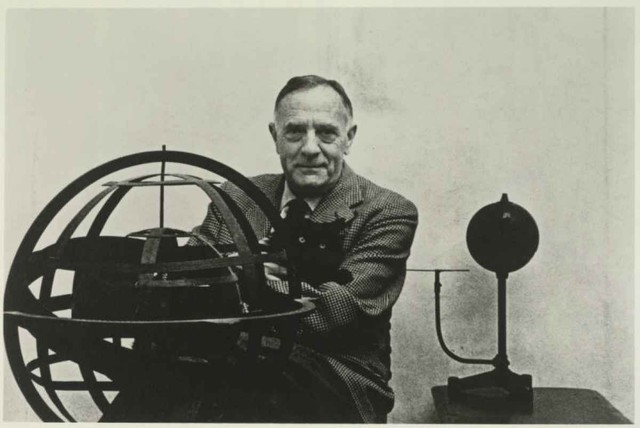This statement is fully consistent with Einstein's description of general relativity, and resolves the contradiction between Einstein's field theory and absolute space-time theory, thus adding a major and important piece of evidence to the model. the big bang universe, showing that the universe began with a singularity explosion, the expansion never stopping.

To make it easier to imagine, it is possible to imagine that the expansion of the universe is like when we bake bread, bread is time and space, when baked bread will expand and raisins inside. In a galaxy-like bread, the raisins don't move at all, but they get further apart as the bread expands.

As space expands, the galaxies will become more and more separated, seemingly moving away from each other. The expansion of the universe is only the expansion of space-time, not the motion of objects with mass, so it is not limited by the speed of light objects.

How is the expansion rate of the universe calculated?

The calculation of the expansion rate of the universe is based on Hubble's law. As mentioned earlier, V represents the total velocity, H represents the Hubble constant, and D represents the actual distance. According to this formula, the Hubble constant must first be known, then the data can be substituted for calculation.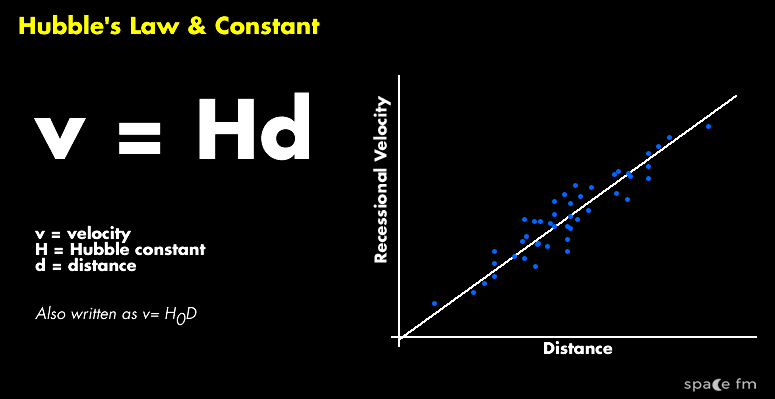To get the correct Hubble constant, over the decades many astronomers have tried a lot of measurement work and obtained some representative data:

In 2006, the Marshall Space Flight Center used the Chandra X-ray telescope to obtain a reading of 77km/s, with an error of about 15%; in 2009, NASA obtained a result of 74.2 ± 3.6km/s based on the measurement of a supernova; In 2013, the ESA result based on the Planck satellite measurement was 67.8 ± 0.77km/s; in 2019, German scientists used the gravitational lensing effect to get a result of 82.4km/s.

Measurement data by each method are not completely consistent, even with large differences. The age and expansion rate of the universe calculated from different data are different. We will therefore compromise these data and get the mean: (67.8 + 77 + 74.2 + 82.4) /4=75.35km.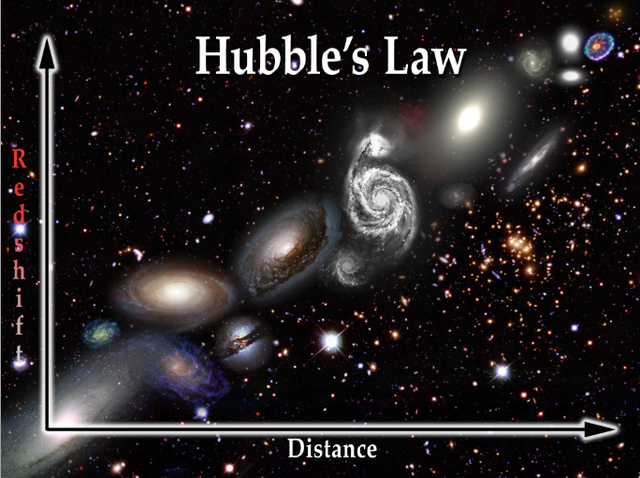Meaning, at a distance of 3.26 million light-years from us, the speed of the galaxy leaving us is about 75.35km/s. Under this compromise, the Hubble constant is used to calculate the expansion rate of the universe. According to the isotropic principle, the farther away, the faster, and the principle that is proportional to the distance, the rate of retreat of galaxies relative to us at any distance can be calculated.

For example, 3.26 million light years away is 75.35km/s; which is 100 million light years away is 2311.35km/s.

The radius of our observable universe is 46.5 billion light-years, which means that the galaxy farthest from us had a speed of 1074777.75km/s as it moved away from us. This is about 3.58 times the speed of light, which is why the so-called universe is expanding faster than the speed of light.

NEW TECHNOLOGY ELECTRONIC REPORT
Responsible agency: Union of Science and High-Tech Production and Telecommunications (HTI) - Vietnam Academy of Science and Technology
Editor in chief: Vo Tran
Operate and exploit advertising by iNoo Vietnam Media and Technology Joint Stock Company.

CONTACT
357A Nguyen Thi Dinh, TT. Tan Phu, Dong Nai.
Tel: (+84) 818.337.007/5 Fax: 818.337.007
Email: Info@azwinn.com